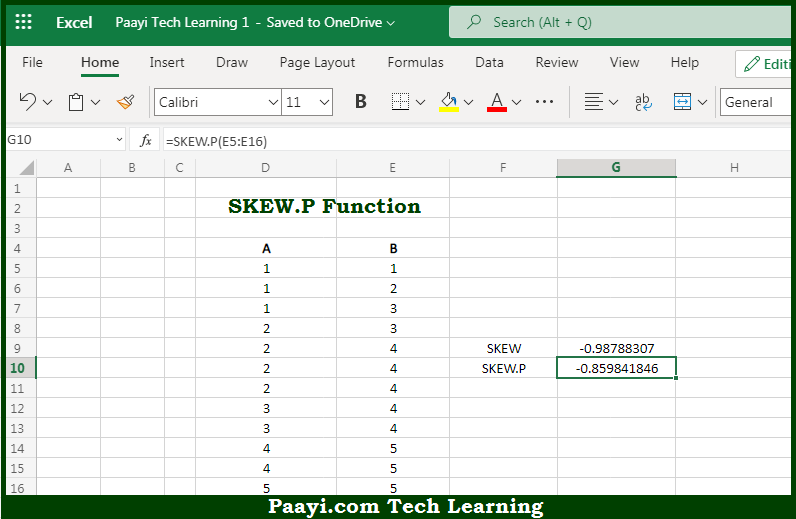# Learn How to Use Microsoft Excel SKEW.P Function

Written by | 0 Comments | 691 Views

In this article, you will learn how to use the Microsoft Excel SKEW.P function and its prime function in Microsoft Excel. You will also get to know the Microsoft Excel SKEW.P function return value and syntax with the help of some examples.

Microsoft Excel SKEW.P Function

The main purpose of the Microsoft Excel SKEW.P function is to get the skewness of the distribution based on population. That implies, with the help of the SKEW.P function you can able to return the skewness of a distribution based on the population, which is a measure of symmetry. A positive result indicates a distribution that tails off to the right. A negative result means a distribution that tails off to the left. So, with the help of the SKEW.P function, you can able to get the skewness of the distribution.

Return Value of SKEW.P Function

The return value will be the positive or negative value.

Syntax of SKEW.P Function

=SKEW.P(number1, [number2], ...)

Where the arguments:

• number1: This is the range or reference that contains numeric values.
• number2: This is the range or reference that contains numeric values (optional).

## How to Use Microsoft Excel SKEW.P Function?So we know that Microsoft Excel SKEW.P function you can able to get the skewness of the distribution based on population. That implies, with the help of the SKEW.P function you can able to return the skewness of a distribution based on the population, which is a measure of symmetry. A positive result indicates a distribution that tails off to the right. A negative result means a distribution that tails off to the left. So, with the help of the SKEW.P function, you can able to get the skewness of the distribution.